# Basis maths-linear algebra-Q17

+1 vote

Let P be a matrix of order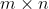and Q be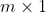column vector (with real entries). Consider the equation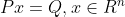admits a unique solution, then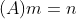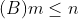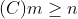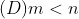asked May 15
reshown May 16

+1 vote

Let has rank then there exists a unique solution in two cases:

For example,

(1) Let here

(2) Let here

Combining the above two equations

we getanswered Jun 11 by (112,390 points)